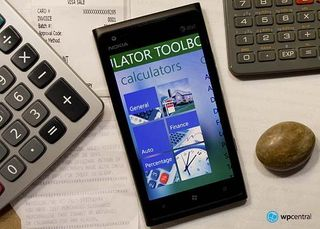# Windows Phone Review: Calculator ToolboxCalculator Toolbox is... well... a calculator app for your Windows Phone. And to call it a calculator app is a mild understatement.

Beyond doing your basic arithmetic Calculator Toolbox throws in six categories that hold thirty four calculators. There are two versions of Calculator Toolbox for you to choose from. A freebie version that has ads and an ad free version that is running \$.99. If you need more than your basic calculator, Calculator Toolbox is worth checking out.

When you first launch Calculator Toolbox, you will quickly realize this isn't your typical calculator app. Calculator Toolbox makes use of the Windows Phone metro design by laying out all the tools in a clean, attractive panoramic style.

You have a page dedicated to your favorite calculators, a page listing the six categories of calculators, a page listing all the converters available (twelve in all), and an about page for the developer.

Within each category you'll find a listing (with description) for each calculator. Asides from your basic calculator, Calculator Toolbox has the follow additional calculators available.

• Tip/Split Calculator
• Discount Calculator
• Debt To Income Ratio Calculator
• Age As Of Calculator
• Adult BMI (Body Mass Index) Calculator
• VAT/Sales Tax Calculator
• Nav S-T-D Calculator
• Six Mortgage Calculators
• Six Auto Calculators
• Three Finance Calculator
• Four Percentage Calculators
• Seven Date and Time Calculators

Not to be left out, here are the converters included with Calculator Toolbox.

• Angle
• Area
• Data
• Data Rate
• Fuel Economy
• Length
• Power
• Speed
• Temperature
• Time
• Volume
• Weight

Once you find your calculator of choice, you'll have a variety of screens (dependent on the calculation) where you enter all your variables and hit the calculate button. From the calculator views you can access the settings(color patterns, tax rates, etc) and a share button to share your calculations with friends, family or co-workers.

Calculator Toolbox has Live Tile support for the Tip, Discount, BMI, Age, DTI Ratio, VAT/Sales Tax, Mortgage, Auto Loan and Auto Lease calculators.

When all the variables are factored, the sum value of the Calculator Toolbox isn't too shabby. The entire app makes the abacus pale in comparison. The interface is easy to navigate around, there are tons of calculators to help you through your daily grind, and the converters can come in handy as well.

Calculator Toolbox Free is an ad-supported version (ads only show up on the calculator view) and you can find the free version here at the Windows Phone Marketplace. If you prefer an ad-free version of Calculator Toolbox, that version is running \$.99 and you can find it here at the Marketplace.